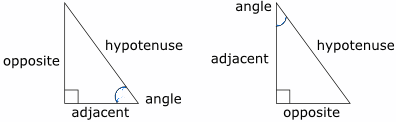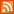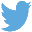Bucaro TecHelpHTTPS Encryption not required because no account numbers orpersonal information is ever requested or accepted by this siteCustom Search# Java Script Math.cos Method

Cosine is a trigonometric function. Based on a right triangle. It's the ratio of the length of the adjacent side to the length of the hypotenuse.In identifying the sides of a right triangle, the side next to the angle for which we are using the trigonometric function is called the adjacent side. The side opposite that angle is called the opposite side.

If you know an angle and the length of the side adjacent to the angle, you can use the cosine of the angle to calculate the length of the hypotenuse.

length of hypotenuse = length of adjacent/cosine of angle

Example

```angle = 35 degrees
opposite side = 8
```

Java Script Using Math.cos Method

```<script type="text/javascript">

var angle = 35;

var hypotenuse = adjacent/Math.cos(35 * Math.PI/180);

 .menubtn { margin-top:10px; margin-left:50px; width:220px; opacity:1; border-style:solid; border-color:#009000; background-color:#66ff66; transition-property: opacity; transition-duration: 1s; transition-timing-function: ease-out; } .menubtn:hover { opacity:1; background-color:#e5ff23; } Menu - More Java Script Quick ReferenceRSS FeedFollow @Stephen Bucaro